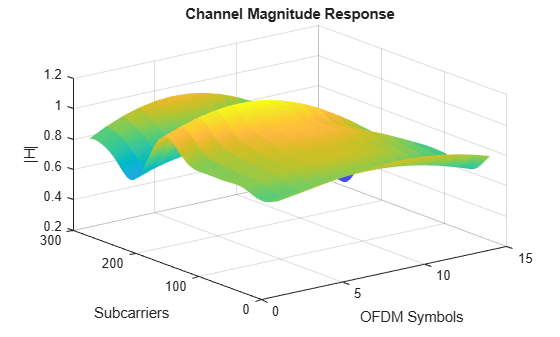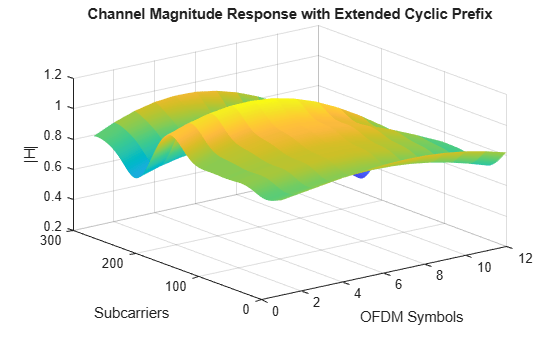# nrPerfectChannelEstimate

Perfect channel estimation

## Syntax

``h = nrPerfectChannelEstimate(carrier,pathGains,pathFilters)``
``h = nrPerfectChannelEstimate(pathGains,pathFilters,nrb,scs,initialNSlot)``
``h = nrPerfectChannelEstimate(___,toffset)``
``h = nrPerfectChannelEstimate(___,toffset,sampleTimes)``
``h = nrPerfectChannelEstimate(___,cpl)``
``h = nrPerfectChannelEstimate(___,Name,Value)``

## Description

````h = nrPerfectChannelEstimate(carrier,pathGains,pathFilters)` performs perfect channel estimation. The function first reconstructs the channel impulse response from the channel path gains `pathGains` and the path filter impulse response `pathFilters`. The function then performs orthogonal frequency division multiplexing (OFDM) demodulation. `carrier` specifies the parameters for the OFDM demodulation.```

example

````h = nrPerfectChannelEstimate(pathGains,pathFilters,nrb,scs,initialNSlot)` performs the OFDM demodulation for `nrb` number of resource blocks with subcarrier spacing `scs` and initial slot number `initialNSlot`.```

example

````h = nrPerfectChannelEstimate(___,toffset)` specifies the timing offset in addition to the input arguments in any of the previous syntaxes. The timing offset indicates the OFDM demodulation starting point on the reconstructed waveform.```
````h = nrPerfectChannelEstimate(___,toffset,sampleTimes)` specifies the sample times of the channel snapshots in addition to the input arguments in the previous syntax.```
````h = nrPerfectChannelEstimate(___,cpl)` specifies the cyclic prefix length in addition to the input arguments in any of the previous syntaxes that do not include the `carrier` input.```
````h = nrPerfectChannelEstimate(___,Name,Value)` specifies options by using one or more name-value pair arguments in addition to the input arguments in any of the previous syntaxes.```

## Examples

collapse all

Define a channel configuration structure using an `nrTDLChannel` System object. Use delay profileTDL-C from TR 38.901 Section 7.7.2.

```SR = 7.68e6; tdl = nrTDLChannel; tdl.DelayProfile = 'TDL-C'; tdl.DelaySpread = 100e-9; tdl.MaximumDopplerShift = 300; tdl.SampleRate = SR;```

Create a random waveform with a duration of 1 subframe.

```T = SR*1e-3; tdlInfo = info(tdl); Nt = tdlInfo.NumTransmitAntennas; in = complex(randn(T,Nt),randn(T,Nt));```

Transmit the input waveform through the channel. Obtain the path filters used in channel filtering.

```[~,pathGains] = tdl(in); pathFilters = getPathFilters(tdl);```

Perform perfect channel estimation using the specified number of blocks, subcarrier spacing, and slot number.

```NRB = 25; SCS = 15; nSlot = 0; hest = nrPerfectChannelEstimate(pathGains,pathFilters,NRB,SCS,nSlot); size(hest)```
```ans = 1×3 300 14 2 ```

Plot the estimated channel magnitude response for the first receive antenna.

```figure; surf(abs(hest(:,:,1))); shading('flat'); xlabel('OFDM Symbols'); ylabel('Subcarriers'); zlabel('|H|'); title('Channel Magnitude Response');```Repeat the channel estimate for extended cyclic prefix.

```hest = nrPerfectChannelEstimate(pathGains,pathFilters,NRB,SCS, ... nSlot,'extended'); size(hest)```
```ans = 1×3 300 12 2 ```

Plot the updated results.

```figure; surf(abs(hest(:,:,1))); shading('flat'); xlabel('OFDM Symbols'); ylabel('Subcarriers'); zlabel('|H|'); title('Channel Magnitude Response with Extended Cyclic Prefix');```Define a channel configuration structure using an `nrCDLChannel` System object. Use delay profile CDL-C from TR 38.901 Section 7.7.1.

``` cdl = nrCDLChannel; cdl.DelayProfile = 'CDL-D'; cdl.DelaySpread = 30e-9; cdl.MaximumDopplerShift = 5;```

Create a random waveform with a duration of 1 subframe.

```SR = 15.36e6; T = SR*1e-3; cdl.SampleRate = SR; cdlInfo = info(cdl); Nt = cdlInfo.NumTransmitAntennas; in = complex(randn(T,Nt),randn(T,Nt));```

Transmit the input waveform through the channel. Obtain the path filters used in channel filtering.

```[~,pathGains,sampleTimes] = cdl(in); pathFilters = getPathFilters(cdl);```

Perform timing offset estimation using the path filter and path gains.

`offset = nrPerfectTimingEstimate(pathGains,pathFilters);`

Perform perfect channel estimation. Use the specified number of blocks, subcarrier spacing, slot number, timing offset, and sample times.

```NRB = 25; SCS = 15; nSlot = 0; hest = nrPerfectChannelEstimate(pathGains,pathFilters,... NRB,SCS,nSlot,offset,sampleTimes); size(hest)```
```ans = 1×4 300 14 2 8 ```

Plot the estimated channel magnitude response for the first receive antenna.

```figure; surf(abs(hest(:,:,1))); shading('flat'); xlabel('OFDM Symbols'); ylabel('Subcarriers'); zlabel('|H|'); title('Channel Magnitude Response');```## Input Arguments

collapse all

Carrier configuration parameters for a specific OFDM numerology, specified as an `nrCarrierConfig` object. Only these object properties are relevant for this function.

Number of RBs in the carrier resource grid, specified as an integer from 1 to 275. The default value of `52` corresponds to the maximum number of RBs of a 10 MHz carrier with 15 kHz SCS.

Data Types: `double`

Subcarrier spacing in kHz, for all channels and reference signals of the carrier, specified as `15`, `30`, `60`, `120`, or `240`.

Data Types: `double`

Slot number, specified as a nonnegative integer. You can set `NSlot` to a value larger than the number of slots per frame. For example, you can set this value using transmission loop counters in a MATLAB® simulation. In this case, you may have to ensure that the property value is modulo the number of slots per frame in a calling code.

Data Types: `double`

Cyclic prefix length, specified as one of these options.

• `'normal'` — Use this value to specify normal cyclic prefix. This option corresponds to 14 OFDM symbols in a slot.

• `'extended'` — Use this value to specify extended cyclic prefix. This option corresponds to 12 OFDM symbols in a slot. For the numerologies specified in TS 38.211 Section 4.2, extended cyclic prefix length applies for only 60 kHz subcarrier spacing.

Data Types: `char` | `string`

Channel path gains of the fading process, specified as an NCS-by-NP-by-NT-by-NR complex matrix, where:

• NCS is the number of channel snapshots.

• NP is the number of paths.

• NT is the number of transmit antennas.

• NR is the number of receive antennas.

Data Types: `single` | `double`
Complex Number Support: Yes

Path filter impulse response, specified as an NH-by-NP real matrix, where:

• NH is the number of impulse response samples.

• NP is the number of paths.

Each column of the matrix contains the filter impulse response for each path of the delay profile.

Data Types: `double`

Number of resource blocks, specified as an integer from 1 to 275.

Data Types: `double`

Subcarrier spacing in kHz, specified as `15`, `30`, `60`, `120`, or `240`.

Data Types: `double`

Initial slot number, 0-based, specified as a nonnegative integer. The function selects the appropriate cyclic prefix length for the OFDM demodulation based on the value of `initialNSlot` modulo the number of slots per subframe.

Data Types: `double`

Timing offset in samples, specified as a nonnegative integer. The timing offset indicates the OFDM demodulation starting point on the reconstructed waveform. The offset accounts for propagation delays, which is essential when obtaining the perfect estimate of the channel seen by a synchronized receiver. `toffset` defaults to the value `nrPerfectTimingEstimate``(pathGains,pathFilters)` when not specified as an input argument.

Data Types: `double`

Sample times of channel snapshots, specified as an NCS-by-1 column vector of nonnegative real numbers. `sampleTimes` specifies the time of occurrence of each channel snapshot. The number of channel snapshots, NCS, is identical to the first dimension of `pathGains`. When not specified, `sampleTimes` defaults to an NCS-by-1 vector of times starting at zero with sampling rate used for the OFDM modulation of the number of resource blocks `nrb` and subcarrier spacing `scs`. Ensure that the channel snapshots span at least one slot. The function performs channel estimation for each complete slot.

Data Types: `double`

Cyclic prefix length, specified as one of these options:

• `'normal'` — Use this value to specify normal cyclic prefix. This option corresponds to 14 OFDM symbols in a slot.

• `'extended'` — Use this value to specify extended cyclic prefix. This option corresponds to 12 OFDM symbols in a slot. For the numerologies specified in TS 38.211 Section 4.2, the extended cyclic prefix length only applies to 60 kHz subcarrier spacing.

Note

• If you specify the `carrier` input, use the `CyclicPrefix` property of the `carrier` input to specify the cyclic prefix length. You cannot use the `cpl` input together with the `carrier` input.

• If you specify the cyclic prefix length with the `'CyclicPrefix'` name-value pair argument, you cannot use the `cpl` input.

Data Types: `char` | `string`

### Name-Value Arguments

Specify optional comma-separated pairs of `Name,Value` arguments. `Name` is the argument name and `Value` is the corresponding value. `Name` must appear inside quotes. You can specify several name and value pair arguments in any order as `Name1,Value1,...,NameN,ValueN`.

Example: `'CyclicPrefixFraction',0.75` specifies the start location for demodulation relative to the cyclic prefix length.

Cyclic prefix length, specified as the comma-separated pair consisting of `'CyclicPrefix'` and one of these values:

• `'normal'` — Use this value to specify normal cyclic prefix. This option corresponds to 14 OFDM symbols in a slot.

• `'extended'` — Use this value to specify extended cyclic prefix. This option corresponds to 12 OFDM symbols in a slot. For the numerologies specified in TS 38.211 Section 4.2, the extended cyclic prefix length only applies to 60 kHz subcarrier spacing.

Note

• If you specify the `carrier` input, use the `CyclicPrefix` property of the `carrier` input to specify the cyclic prefix length. You cannot use this name-value pair argument together with the `carrier` input.

• If you specify the cyclic prefix length with the `cpl` input, you cannot use this name-value pair argument.

Data Types: `char` | `string`

Number of fast Fourier transform (FFT) points, specified as the comma-separated pair consisting of `'Nfft'` and a nonnegative integer greater than 127 or `[]`. The value you specify must result in integer-valued cyclic prefix lengths and a maximum occupancy of 100%. The occupancy is defined as the value of (12 × NRB)/`Nfft`, where NRB is the number of resource blocks.

If you do not specify this input, or if you specify `'Nfft',[]`, the function sets an integer value greater than 127 as a default value for this input. The actual default value depends on other input values.

• If you do not specify the `SampleRate` input, or if you specify `'SampleRate',[]`, the function sets `Nfft` satisfying these conditions.

• `Nfft` is an integer power of 2.

• `Nfft` results in a maximum occupancy of 85%.

• If you specify the `SampleRate` input, the function sets `Nfft` satisfying these conditions.

• `Nfft` results in integer-valued cyclic prefix lengths.

• `Nfft` maximises the value of `gcd` (`Nfft` × SCS, `SampleRate`), where SCS is specified by the `carrier``.SubcarrierSpacing` property or the `scs` input.

Data Types: `double`

Waveform sample rate, specified as the comma-separated pair consisting of `'SampleRate'` and either a positive scalar or `[]`.

If you do not specify this input, or if you specify `'SampleRate',[]`, then the function sets this input to the value of Nfft × SCS.

Data Types: `double`

Fast Fourier transform (FFT) window position within the cyclic prefix, specified as the comma-separated pair consisting of `'CyclicPrefixFraction'` and a scalar in the interval [0, 1].

The value that you specify indicates the start location for OFDM demodulation relative to the beginning of the cyclic prefix.

Data Types: `double`

## Output Arguments

collapse all

Perfect channel estimate, returned as an NSC-by-NSYM-by-NR-by-NT complex array, where:

• NSC is the number of subcarriers.

• NSYM is the number of OFDM symbols.

• NR is the number of receive antennas.

• NT is the number of transmit antennas.

`h` inherits its data type from `pathGains`.

Data Types: `double` | `single`

 3GPP TS 38.211. “NR; Physical channels and modulation.” 3rd Generation Partnership Project; Technical Specification Group Radio Access Network.